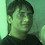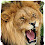## How to find AC Motor/Induction Motor Current,Synchronous Speed

To calculate motor parameters is very important for deciding the rating of motor and its suitability for the load. In this first section we will study the basics formula for a AC induction motor.
1) To find out the synchronous speed apply the below formula
Ns= 120 x F/P

Where Ns is synchronous speed , F is frequency and P is number of poles of motor.

2) To find out the horse power of a motor when rpm and torque are known use the below formula.

HP = rpm x T (torque)/5252(constant)

This above formula is very use full if the data on the nameplate of motor is not available. We can find HP or KW by using above equation

1 HP = 746 watt.

3) There is also an another way to find rating of motor if the voltage and current , efficiency of motor are known
HP = I X E X Eff./746

This formula proves help full and give the accurate rating of motor.

Inversely from the above equation you can find current of motor also if all other parameters are known to you.
Below is a table which will depict an example as how to calculate.

Horsepower Formulas

 Horsepower Formulas To Find Use Formula Example Given Find Solution HP HP = I X E X Eff. 746 240V, 20A, 85% Eff. HP HP = 240V x 20A x 85% 746HP=5.5 I I = HP x 746 E X Eff x PF 10HP, 240V,90% Eff., 88% PF I I = 10HP x 746 240V x 90% x 88%I = 39 A

In the next section we will discuss further about the more motor parameters and the equations to find them.
Subscribe to Automation-Talk by Email.

1.Thanks good post

2.yes a good one ...it helped me to understand a lot

3.is motor rated to input power or output HP??

4.Motor Rating is for its Output HP.